# 扭曲矩形管管内传热与压降特性的数值研究Numerical Study of Heat Transfer and Pressure Drop Characteristics in Twisted Rectangular Tube

DOI: 10.12677/APP.2021.112012, PDF, HTML, XML, 下载: 91  浏览: 572  科研立项经费支持

Abstract: The characteristics of heat transfer and pressure drop in the twisted rectangular tubes are numerically studied by CFD method. Some sets of data about heat transfer and pressure drop characteristics of twisted rectangular tube with different geometric parameters under the Reynolds number 5000~40,000 are obtained. In addition, the mechanism heat transfer enhancement was analyzed by data comparison. The results show that: in turbulent flow, the friction coefficient decreased with the increase of the aspect ratio, and the Nusselt number first increased and then decreased with the increase of the aspect ratio. The Nusselt number reached the maximum when the twist ratio was 0.4. In turbulent flow, the friction coefficient decreased with the increase of the torque, and reached the maximum value when the twisted pitch length was 200 mm, where the twisted rectangular tube had high Nusselt number. The secondary flow in the twisted rectangular tube promotes the heat exchange between the fluid inside and outside the thermal boundary layer, thus improving the comprehensive heat transfer performance of the twisted rectangular tube.

1. 引言

2. 数值模型

2.1. 物理模型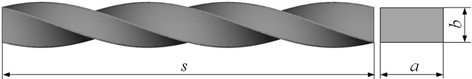Figure 1. Physical model of twisted rectangular tubeTable 1. System resulting data of standard experiment

2.2. 控制方程

$\frac{\partial \rho }{\partial t}+\frac{\partial \left(\rho {\mu }_{j}\right)}{\partial {x}_{j}}=0$ (1)

$\rho \frac{\partial k}{\partial t}+\rho \frac{\partial \left({\mu }_{j}k\right)}{\partial {x}_{j}}=\frac{\partial p}{\partial {x}_{j}}\left[\left(\mu +\frac{{\mu }_{i}}{{\sigma }_{k}}\right)\frac{\partial k}{\partial {x}_{j}}\right]+{\eta }_{t}\frac{\partial {\mu }_{i}}{\partial {x}_{j}}\left(\frac{\partial {\mu }_{i}}{\partial {x}_{j}}+\frac{\partial {\mu }_{j}}{\partial {x}_{i}}\right)-\rho \epsilon$ (2)

$\rho \frac{\partial \epsilon }{\partial t}+\rho \frac{\partial \left({\mu }_{j}\epsilon \right)}{\partial {x}_{j}}=\frac{\partial }{\partial {x}_{j}}\left[\left(\mu +\frac{{\mu }_{t}}{{\sigma }_{\epsilon }}\right)\frac{\partial \epsilon }{\partial {x}_{j}}\right]+{c}_{1}\rho S\epsilon -{c}_{2}\rho \frac{{\epsilon }^{2}}{k+\sqrt{v\epsilon }}$ (3)

${c}_{1}=\mathrm{max}\left[0.43,\frac{\eta }{\eta +5}\right];\text{\hspace{0.17em}}\eta =\frac{Sk}{\epsilon };\text{\hspace{0.17em}}=\sqrt{2{S}_{i,j}{S}_{i,j}}；{S}_{i,j}=\frac{1}{2}\left(\frac{\partial {\mu }_{i}}{\partial {x}_{j}}+\frac{\partial {\mu }_{j}}{\partial {x}_{i}}\right)$ (4)

${c}_{2}=1.9;\text{\hspace{0.17em}}{\mu }_{l}=\frac{{c}_{\mu }\rho {k}^{2}}{\epsilon };\text{\hspace{0.17em}}{\sigma }_{k}=1.0;\text{\hspace{0.17em}}{\sigma }_{\epsilon }=1.2$ (5)

$\rho \frac{\partial T}{\partial t}+\rho \frac{\partial \left({u}_{i}T\right)}{\partial {x}_{i}}=\frac{\lambda }{{c}_{p}}\frac{{\partial }^{2}T}{\partial {x}_{i}^{2}}$ (6)

2.3 网格划分及边界条件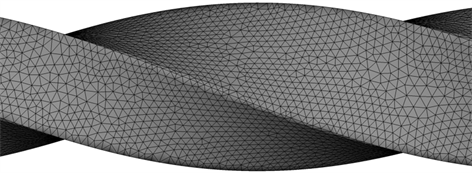Figure 2. Meshing of twisted tube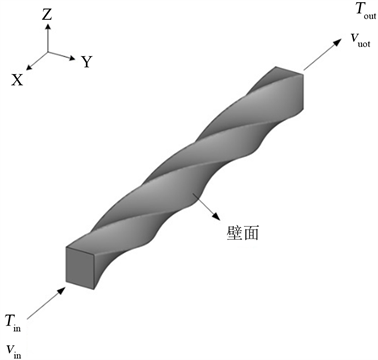Figure 3. 3D model of twisted rectangular tube

3. 数据处理

$\mathrm{Re}=\frac{\rho vd}{\mu }$ (7)

$v=\frac{{q}_{m}}{\rho ab}$ (8)

$d=\frac{2ab}{a+b}$ (9)

$f=\frac{2\Delta Pd}{\rho s{v}^{2}}$ (10)

$Nu=\frac{qd}{\left({T}_{w}-{T}_{b}\right)\lambda }$ (11)

$\eta =\left(Nu/N{u}_{0}\right)/{\left(f/{f}_{0}\right)}^{1/3}$ (12)

4. 模型计算结果分析

4.1. 数值方法可靠性验证

1) 网格独立性分析Figure 4. Grid number of twisted rectangular tube

2) 模型验证

4.2. 长宽比对扭曲矩形管性能的影响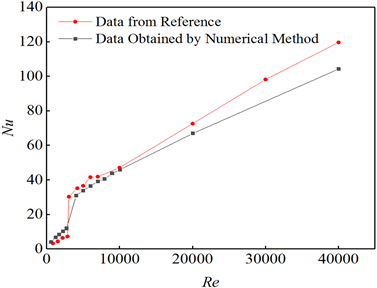Figure 5. Comparison of Nu between numerical method and relative literature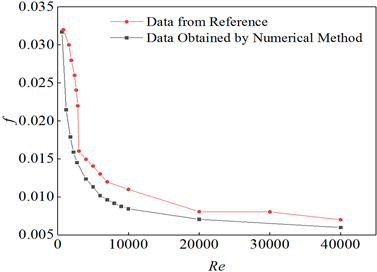Figure 6. Comparison of friction coefficient between numerical method and relative literature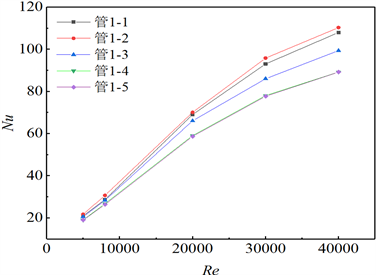Figure 7. Wall Nu number under different Re number at turbulent flow stage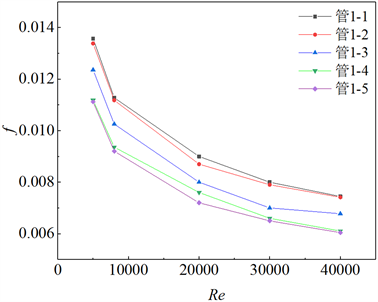Figure 8. Wall friction coefficient under different Re number at turbulent flow stage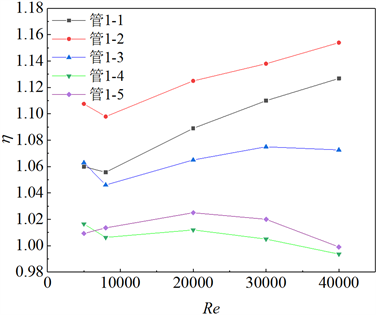Figure 9. Comprehensive performance of heat exchange tube at turbulent flow stage

4.3. 扭距对扭曲矩形管性能的影响Figure 10. Wall Nu number under different Re number at turbulent flow stage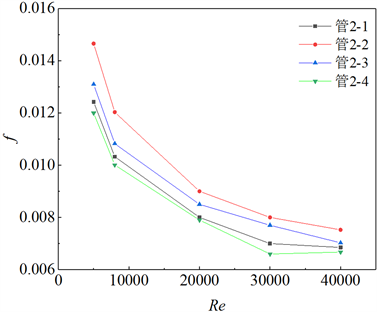Figure 11. Wall friction coefficient under different Re number at turbulent flow stage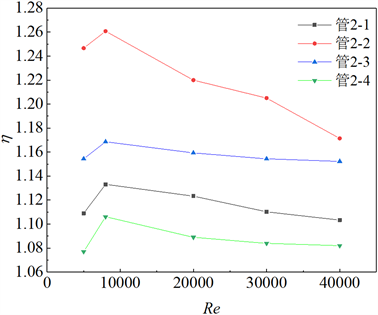Figure 12. Comprehensive performance of heat exchange tube at turbulent flow stage

4.4. 扭曲矩形管管内强化传热机理分析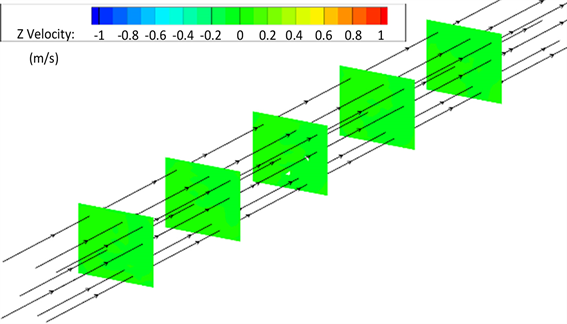Figure 13. Streamlines and velocity distribution contours in Tube 3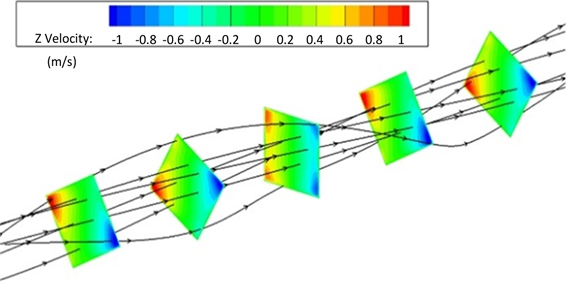Figure 14. Streamlines and velocity contours in Tube 1-5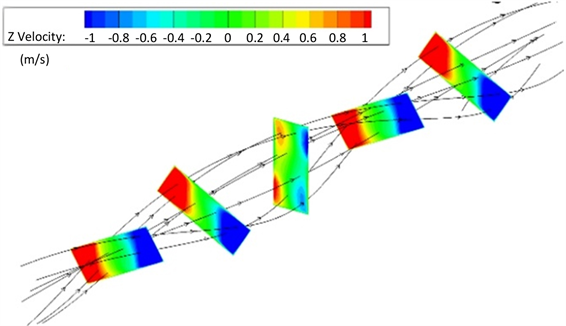Figure 15. Streamlines and velocity contours in Tube 1-2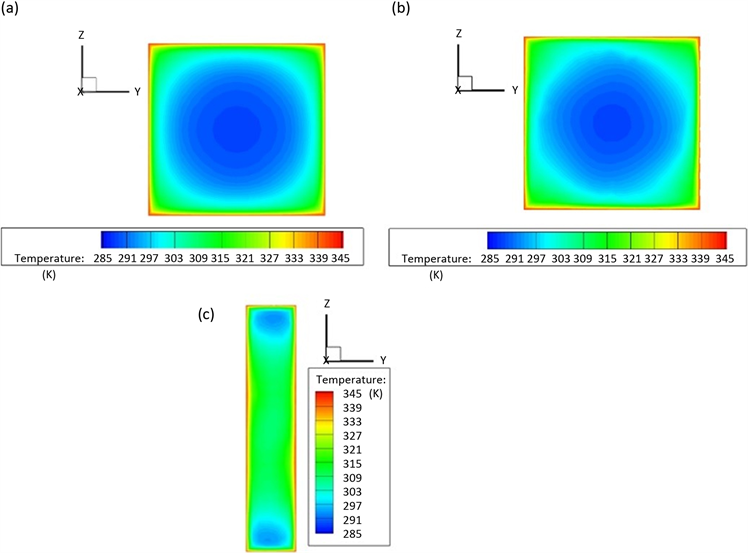Figure 16. (a) Temperature distribution in cross section of Tube 3; (b) Temperature distribution in cross section of Tube 1-5; (c) Temperature distribution in cross section of Tube 1-2

5. 结论

1) 通过SolidWorks软件建立了物理模型，使用CFD相关软件完成了网格划分和管内流体流动的模拟，得出了壁面平均Nu和f，与前人的实验研究结果进行了比对，确定了数值方法的可靠性。

2) 对得到的不同扭曲矩形管的壁面平均Nu和f进行了对比，发现当600 ≤ Re ≤ 40,000时，随着k的增加综合换热性能先增加后减小，在k = 0.4时达到最大值。随着s的增加，综合换热性能先增加后减少，在s = 200 mm时达到最大值。

3) 对扭曲矩形管管内流线、速度场和温度场进行了分析，结果表明二次流的出现改变了换热管横截面速度场分布，进而影响了流动截面的温度分布，实现了强化传热。

“高效节能的换热/反应装备关键技术”产学研合作项目(校企合作)。

  Bishara, F. (2010) Numerical Simulation of Fully Developed Laminar Flow and Heat Transfer in Isothermal Helically Twisted Tubes with Elliptical Cross-Sections. University of Cincinnati, Cincinnati.  高学农, 邹华春, 王端阳, 等. 高扭曲比螺旋扁管的管内传热及流阻性能[J]. 华南理工大学学报(自然科学版), 2008, 36(11): 17-21+26.  Wang, L.B., Tao, W.Q., Wang, Q.W., et al. (2001) Experimental and Numerical Study of Turbulent Heat Transfer in Twisted Square Ducts. Journal of Heat Transfer, 123, 868-877. https://doi.org/10.1115/1.1389464  Todd, L. (1977) Some Comments on Steady, Laminar Flow through Twisted Pipes. Journal of Engineering Mathematics, 11, 29-48. https://doi.org/10.1007/BF01535586  Keun-Shik, C., et al. (1988) Laminar Fluid Flow in a Twisted Elliptic Tube. KSME Journal, 2, 44-51. https://doi.org/10.1007/BF02944075  Yang, S., Zhang, L. and Xu, H. (2011) Experimental Study on Convec-tive Heat Transfer and Flow Resistance Characteristics of Water Flow in Twisted Elliptical Tubes. Applied Thermal En-gineering, 31, 2981-2991. https://doi.org/10.1016/j.applthermaleng.2011.05.030  Manglik, R.M., Patel, P. and Jog, M.A. (2012) Swirl-Enhanced Laminar Forced Convection through Axially Twisted Rectangular Ducts—Part 2, Fluid Flow. Journal of Enhanced Heat Transfer, 19, 423-436. https://doi.org/10.1115/HT2012-58304  吴欣慰, 刘长军, 梁斌, 等. 扭曲扁平管结构对管内传热强化与阻力特性的影响[J]. 化工机械, 2017, 44(5): 547-552.  Patel, P., Manglik, R.M. and Jog, M.A. (2012) Swirl-Enhanced Laminar Forced Convection through Axially Twisted Rectangular Ducts—Part 1, Fluid Flow. Journal of Enhanced Heat Transfer, 19, 423-436. https://doi.org/10.1615/JEnhHeatTransf.v19.i5.30  Dzyubenko, B.V. (1983) Drag in a Heat Exchanger with a Twisted Flow. Journal of Engineering Physics, 44, 237-241. https://doi.org/10.1007/BF00827353  Dzyubenko, B.V. and Dreitser, G.A. (1986) Heat Transfer and Fluid Friction in Bundles of Twisted Tubes. Journal of Engineering Physics, 50, 611-618. https://doi.org/10.1007/BF00871525  Evlev, V., Kalinin, E., Danilov, I., et al. (1982) Heat Transfer in the Turbulent Swirling Flow in a Channel of Complex Shape. Proceedings of the Seventh International Conference, Vol. 3, Munich, 6-10 September 1982, 171-176. https://doi.org/10.1615/IHTC7.1660  刘思宇. 扭曲管管程数值传热模拟[J]. 科技创新导报, 2019, 16(6): 115-117.  张立振. 内螺纹扭曲管换热器强化传热与流阻性能研究及其工程应用[D]: [硕士学位论文]. 上海: 华东理工大学, 2013.  周云龙, 张超, 张立彦, 等. 矩形螺旋通道气液两相流流动和传热特性数值研究[J]. 化工机械, 2016, 43(3): 357-364.  覃文洁, 胡春光, 郭良平, 等. 近壁面网格尺寸对湍流计算的影响[J]. 北京理工大学学报, 2006, 26(5): 388-392.  Bhadouriya, R., Agrawal, A. and Prabhu, S.V. (2015) Experimental and Numerical Study of Fluid Flow and Heat Transfer in a Twisted Square Duct. International Journal of Heat & Mass Transfer, 82, 143-158. https://doi.org/10.1016/j.ijheatmasstransfer.2014.11.054  韩文娟. 关于U形管式换热器温度场的数值模拟研究[J]. 应用物理, 2020, 10(11): 476-482.  王超. 冷却条件下超临界CO2在突扩管中流动及其换热的数值模拟[J]. 应用物理, 2015, 5(12): 216-222.  甄嘉鹏. 多层介质传热的计算模拟[J]. 应用物理, 2019, 9(1): 7-12.